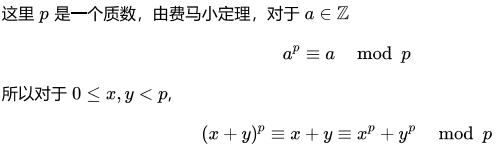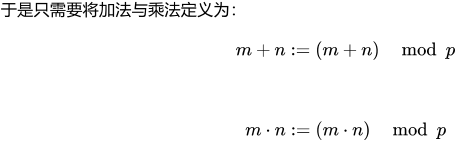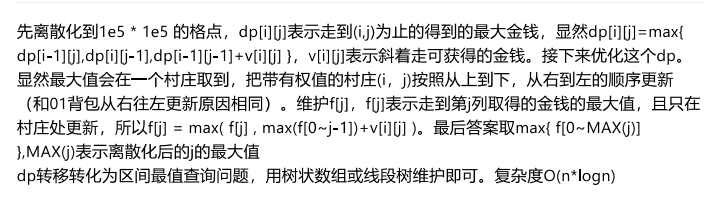# 1001

Time Limit: 2000/1000 MS (Java/Others)    Memory Limit: 65536/65536 K (Java/Others)
Total Submission(s): 1011    Accepted Submission(s): 313

Problem Description

The Power Cube is used as a stash of Exotic Power. There are n cities numbered 1,2,…,n where allowed to trade it. The trading price of the Power Cube in the i-th city is ai dollars per cube. Noswal is a foxy businessman and wants to quietly make a fortune by buying and reselling Power Cubes. To avoid being discovered by the police, Noswal will go to the i-th city and choose exactly one of the following three options on the i-th day:

1. spend ai dollars to buy a Power Cube
2. resell a Power Cube and get ai dollars if he has at least one Power Cube
3. do nothing

Obviously, Noswal can own more than one Power Cubes at the same time. After going to the n cities, he will go back home and stay away from the cops. He wants to know the maximum profit he can earn. In the meanwhile, to lower the risks, he wants to minimize the times of trading (include buy and sell) to get the maximum profit. Noswal is a foxy and successful businessman so you can assume that he has infinity money at the beginning.

Input

There are multiple test cases. The first line of input contains a positive integer T (T≤250), indicating the number of test cases. For each test case:
The first line has an integer n. (1≤n≤105)
The second line has n integers a1,a2,…,an where ai means the trading price (buy or sell) of the Power Cube in the i-th city. (1≤ai≤109)
It is guaranteed that the sum of all n is no more than 5×105.

Output

For each case, print one line with two integers —— the maximum profit and the minimum times of trading to get the maximum profit.

Sample Input

3
4
1 2 10 9
5
9 5 9 10 5
2
2 1

Sample Output

16 4
5 2
0 0

Hint

In the first case, he will buy in 1, 2 and resell in 3, 4. profit = - 1 - 2 + 10 + 9 = 16 In the second case, he will buy in 2 and resell in 4. profit = - 5 + 10 = 5 In the third case, he will do nothing and earn nothing. profit = 0

Source

2018中国大学生程序设计竞赛 - 网络选拔赛

Recommend

chendu   |   We have carefully selected several similar problems for you:  6447 6446 6445 6444 6443

### 分析：

#include<iostream>
#include <cstdio>
#include<algorithm>
#include <cstring>
#include <string>
#include <cmath>
#include <vector>
#include <set>
#include <queue>
#include <map>
#include <stack>
#include <bitset>

using namespace std;
typedef pair<int,int> P;
typedef long long ll;

const int maxn = 1e5 + 7;
const ll mod = 1e9 + 7;
map<int,int> vis;

int main() {
int T;
scanf("%d", &T);
while(T--) {
int n;
scanf("%d", &n);
priority_queue<int, vector<int>, greater<int> > qu;
while(!qu.empty()) qu.pop();
ll ans = 0, cnt = 0;
vis.clear();
for(int i = 1; i <= n; ++i) {
int x; scanf("%d", &x);
if(qu.empty() || qu.top() >= x) {
qu.push(x);
}
else {
cnt++;
int t = qu.top(); qu.pop();
ans += (x-t);
if(vis[t] > 0) {
cnt--;
vis[t]--;
}
qu.push(x); qu.push(x);
vis[x]++;
}
}
printf("%lld %lld\n", ans, cnt*2);
}
return 0;
}


# Dream

Time Limit: 12000/6000 MS (Java/Others)    Memory Limit: 65536/65536 K (Java/Others)
Total Submission(s): 954    Accepted Submission(s): 163
Special Judge

Problem Description

Freshmen frequently make an error in computing the power of a sum of real numbers, which usually origins from an incorrect equation (m+n)p=mp+np, where m,n,p are real numbers. Let's call it Beginner's Dream''.

For instance, (1+4)2=52=25, but 12+42=17≠25. Moreover, 9+16−−−−−√=25−−√=5, which does not equal 3+4=7.

Fortunately, in some cases when p is a prime, the identity

(m+n)p=mp+np

holds true for every pair of non-negative integers m,n which are less than p, with appropriate definitions of addition and multiplication.

You are required to redefine the rules of addition and multiplication so as to make the beginner's dream realized.

Specifically, you need to create your custom addition and multiplication, so that when making calculation with your rules the equation (m+n)p=mp+np is a valid identity for all non-negative integers m,n less than p. Power is defined as

ap={1,ap−1⋅a,p=0p>0

Obviously there exists an extremely simple solution that makes all operation just produce zero. So an extra constraint should be satisfied that there exists an integer q(0<q<p) to make the set {qk|0<k<p,k∈Z} equal to {k|0<k<p,k∈Z}. What's more, the set of non-negative integers less than p ought to be closed under the operation of your definitions.

Hint

Hint for sample input and output:
From the table we get 0+1=1, and thus (0+1)2=12=1⋅1=1. On the other hand, 02=0⋅0=0, 12=1⋅1=1, 02+12=0+1=1.
They are the same.

Input

The first line of the input contains an positive integer T(T≤30) indicating the number of test cases.

For every case, there is only one line contains an integer p(p<210), described in the problem description above. p is guranteed to be a prime.

Output

For each test case, you should print 2p lines of p integers.

The j-th(1≤j≤p) integer of i-th(1≤i≤p) line denotes the value of (i−1)+(j−1). The j-th(1≤j≤p) integer of (p+i)-th(1≤i≤p) line denotes the value of (i−1)⋅(j−1).

Sample Input

1
2

Sample Output

0 1
1 0
0 0
0 1

Source

2018中国大学生程序设计竞赛 - 网络选拔赛

Recommend

chendu   |   We have carefully selected several similar problems for you:  6447 6446 6445 6444 6443

### 分析：#include<cstdio>
using namespace std;
int main()
{
int T;
scanf("%d",&T);
while(T--){
int p;
scanf("%d",&p);
for(int i=1;i<=p;i++)
for(int j=1;j<=p;j++)
{
printf("%d",(i+j-2)%p);
if(p==j)    printf("\n");
else printf(" ");
}
for(int i=1;i<=p;i++)
for(int j=1;j<=p;j++)
{
printf("%d",((i-1)*(j-1))%p);
if(p==j)    printf("\n");
else printf(" ");
}
}
return 0;
}


# Find Integer

Time Limit: 2000/1000 MS (Java/Others)    Memory Limit: 65536/65536 K (Java/Others)
Total Submission(s): 819    Accepted Submission(s): 155
Special Judge

Problem Description

people in USSS love math very much, and there is a famous math problem .

give you two integers n,a,you are required to find 2 integers b,c such that an+bn=cn.

Input

one line contains one integer T;(1≤T≤1000000)

next T lines contains two integers n,a;(0≤n≤1000,000,000,3≤a≤40000)

Output

print two integers b,c if b,c exits;(1≤b,c≤1000,000,000);else print two integers -1 -1 instead.

Sample Input

1

2 3

Sample Output

4 5

Source

2018中国大学生程序设计竞赛 - 网络选拔赛

Recommend

chendu   |   We have carefully selected several similar problems for you:  6447 6446 6445 6444 6443

### 分析：

n=0时，无解

n=1时，b=1,c=a+1

n=2时，直角三角形a^2+b^2=c^2整数解的定a公式直求法

a=2n+1
b= n^2+（n+1）^2-1
c= n^2+（n+1）^2

a= 2n
b= n^2 -1
c= n^2+1

n > 2时，由费马大定理可知，

#include<bits/stdc++.h>
using namespace std;
const int maxn=1e9+10;
int main()
{
int T;
scanf("%d",&T);
while(T--){
int n,a;
scanf("%d%d",&n,&a);
if(n==0)
printf("-1 -1\n");
else if(n==1)
printf("1 %d\n",a+1);
else if(n==2)
{
if(a%2)
{
int N=a/2;
int b=N*N+(N+1)*(N+1)-1;
printf("%d %d\n",b,b+1);
}
else
{
int N=a/2;
int b=N*N-1;
printf("%d %d\n",b,b+2);
}
}
else printf("-1 -1\n");
}
return 0;
}


#include<bits/stdc++.h>
using namespace std;
#define ll long long
struct node{
ll b,c;
}aa;
void init(){
memset(aa,0,sizeof(aa));
for(ll i = 3;i<=40000;i++){
for(ll j = 1;j<i;j++){
if(i*i%j==0){
ll x = j;
ll y = i*i/j;
if((x+y)%2==0){
aa[i].c=(x+y)/2;
aa[i].b=(y-x)/2;
break;
}
}
}
}
}
int main(){
init();
int t;
scanf("%d",&t);
while(t--){
ll a,b,c,n;
scanf("%lld%lld",&n,&a);
if(n>2||n==0){
printf("-1 -1\n");
continue;
}
else if(n==1){
printf("1 %lld\n",a+1);
continue;
}
else{
if(aa[a].b){
printf("%lld %lld\n",aa[a].b,aa[a].c);
}
else
printf("-1 -1\n");
}

}
return 0;
}


# Tree and Permutation

Time Limit: 2000/1000 MS (Java/Others)    Memory Limit: 65536/65536 K (Java/Others)
Total Submission(s): 725    Accepted Submission(s): 254

Problem Description

There are N vertices connected by N−1 edges, each edge has its own length.
The set { 1,2,3,…,N } contains a total of N! unique permutations, let’s say the i-th permutation is Pi and Pi,j is its j-th number.
For the i-th permutation, it can be a traverse sequence of the tree with N vertices, which means we can go from the Pi,1-th vertex to the Pi,2-th vertex by the shortest path, then go to the Pi,3-th vertex ( also by the shortest path ) , and so on. Finally we’ll reach the Pi,N-th vertex, let’s define the total distance of this route as D(Pi) , so please calculate the sum of D(Pi) for all N! permutations.

Input

There are 10 test cases at most.
The first line of each test case contains one integer N ( 1≤N≤105 ) .
For the next N−1 lines, each line contains three integer X, Y and L, which means there is an edge between X-th vertex and Y-th of length L ( 1≤X,Y≤N,1≤L≤109 ) .

Output

For each test case, print the answer module 109+7 in one line.

Sample Input

3
1 2
1 2
3 1
3
1 2
1 1
3 2

Sample Output

16
24

Source

2018中国大学生程序设计竞赛 - 网络选拔赛

Recommend

chendu   |   We have carefully selected several similar problems for you:  6447 6446 6445 6444 6443

#include <bits/stdc++.h>
using namespace std;
const int maxn = 1e6 + 10;
const int mod = 1e9 + 7;
int head[maxn], n, cnt, x, y, v;
long long int ans,faz[maxn] = {0, 0, 1}, size[maxn];
struct edge {
int next, to;
long long int v;//边权
} edge[maxn];

void add_edge(int x, int y, int v) {
edge[cnt].to = y;
edge[cnt].v = v;
}

//faz[i]表示(i-1)的阶乘
void fazz() {
for (int i = 3; i <= 1e5 + 2; i++) {
faz[i] = (faz[i - 1] * (i - 1))%mod;
}
}

int dfs(int x, int fa) {
size[x] = 1;
for (int i = head[x]; i; i = edge[i].next) {
int son = edge[i].to, cost = edge[i].v;
if (fa == son) continue;
size[x] += dfs(son, x);
ans = (ans + (size[son] * (n - size[son]))%mod * cost)%mod;
}
return size[x];
}

int main()
{
fazz();
while (scanf("%d", &n) != EOF) {
memset(size, 0, sizeof size);
memset(edge, 0, sizeof edge);
cnt = ans = 0;
for (int i = 0; i < n - 1; i++) {
scanf("%d %d %d", &x, &y, &v);
}

dfs(1, -1);

printf("%lld\n", ((faz[n] * 2)%mod *ans)%mod);
}
return 0;
}

# YJJ's Salesman

Time Limit: 4000/2000 MS (Java/Others)    Memory Limit: 65536/65536 K (Java/Others)
Total Submission(s): 1048    Accepted Submission(s): 350

Problem Description

YJJ is a salesman who has traveled through western country. YJJ is always on journey. Either is he at the destination, or on the way to destination.
One day, he is going to travel from city A to southeastern city B. Let us assume that A is (0,0) on the rectangle map and B (109,109). YJJ is so busy so he never turn back or go twice the same way, he will only move to east, south or southeast, which means, if YJJ is at (x,y) now (0≤x≤109,0≤y≤109), he will only forward to (x+1,y), (x,y+1) or (x+1,y+1).
On the rectangle map from (0,0) to (109,109), there are several villages scattering on the map. Villagers will do business deals with salesmen from northwestern, but not northern or western. In mathematical language, this means when there is a village k on (xk,yk) (1≤xk≤109,1≤yk≤109), only the one who was from (xk−1,yk−1) to (xk,yk) will be able to earn vk dollars.(YJJ may get different number of dollars from different village.)
YJJ has no time to plan the path, can you help him to find maximum of dollars YJJ can get.

Input

The first line of the input contains an integer T (1≤T≤10),which is the number of test cases.

In each case, the first line of the input contains an integer N (1≤N≤105).The following N lines, the k-th line contains 3 integers, xk,yk,vk (0≤vk≤103), which indicate that there is a village on (xk,yk) and he can get vk dollars in that village.
The positions of each village is distinct.

Output

The maximum of dollars YJJ can get.

Sample Input

1
3
1 1 1
1 2 2
3 3 1

Sample Output

3

Source

2018中国大学生程序设计竞赛 - 网络选拔赛

Recommend

chendu   |   We have carefully selected several similar problems for you:  6447 6446 6445 6444 6443

### 分析：#include<bits/stdc++.h>
#define lson rt<<1
#define rson rt<<1|1
#define mme(a,b) memset((a),(b),sizeof((a)))
using namespace std;
typedef unsigned long long LL;
const int N = 2e5 + 7;
const int M = 1e5 + 7;
const int MOD = 1e9 + 7;
const int INF = 0x3f3f3f3f;
int ar[N],br[N];//离散化
int dp[N];
struct lh{//储存1e5个点
int x,y,v;
}op[N];
bool cmp(lh &a,lh &b){
if(a.x!=b.x)return a.x<b.x;
return a.y>b.y;
}
int n;
/**********线段树区间最值**********/
struct lp{
int l, r, sum;
}cw[N<<2];
void push_up(int rt){
cw[rt].sum = max(cw[lson].sum, cw[rson].sum);
}
void build(int l,int r,int rt){
cw[rt].l = l;cw[rt].r = r;cw[rt].sum = 0;
if(l==r){
return;
}
int mid = (l+r)/2;
build(l,mid,lson);build(mid+1,r,rson);
push_up(rt);
}
void update(int p,int c,int rt){
int l = cw[rt].l, r = cw[rt].r,mid = (l+r)/2;
if(l==r){
cw[rt].sum = c;
return;
}
if(p<=mid)update(p,c,lson);
else update(p,c,rson);
push_up(rt);
}
int query(int L,int R,int rt){
int l = cw[rt].l, r = cw[rt].r,mid = (l+r)/2;
if(L<=l&&r<=R){
return cw[rt].sum;
}
if(L>mid)return query(L,R,rson);
else if(R<=mid)return query(L,R,lson);
return max(query(L,mid,lson),query(mid+1,R,rson));
}
/****************/
int main(){
int tim;
scanf("%d", &tim);
while(tim--){
scanf("%d", &n);
for(int i = 0; i < n; ++i){
scanf("%d%d%d",&op[i].x,&op[i].y,&op[i].v);
ar[i] = op[i].x;br[i]=op[i].y;
}
int p = n + 1;
ar[n] = 0;br[n] = 0;
sort(ar,ar+p);
sort(br,br+p);
int a = unique(ar,ar+p)-ar;
int b = unique(br,br+p)-br;
for(int i = 0; i < n; ++i){
op[i].x=lower_bound(ar,ar+a,op[i].x)-ar;
op[i].y=lower_bound(br,br+b,op[i].y)-br;
}
//以上离散化
sort(op,op+n,cmp);
build(0, b, 1);
mme(dp, 0);
for(int i = 0; i < n; ++i){
int flag = op[i].x, j;
for(j = i; j < n; ++j){
if(op[j].x != flag){
break;
}
int tmp = query(0, op[j].y-1, 1) + op[j].v;
if(tmp > dp[op[j].y]){
dp[op[j].y] = tmp;
update(op[j].y, dp[op[j].y], 1);
}
}
i = j - 1;
}
int ans = 0;
for(int i = 0; i <= b; ++i){
ans = max(ans, dp[i]);
}
printf("%d\n", ans);
}
return 0;
}

10-26
08-27135608-26955
10-169960
08-287633
03-251万+
07-042440
09-18106